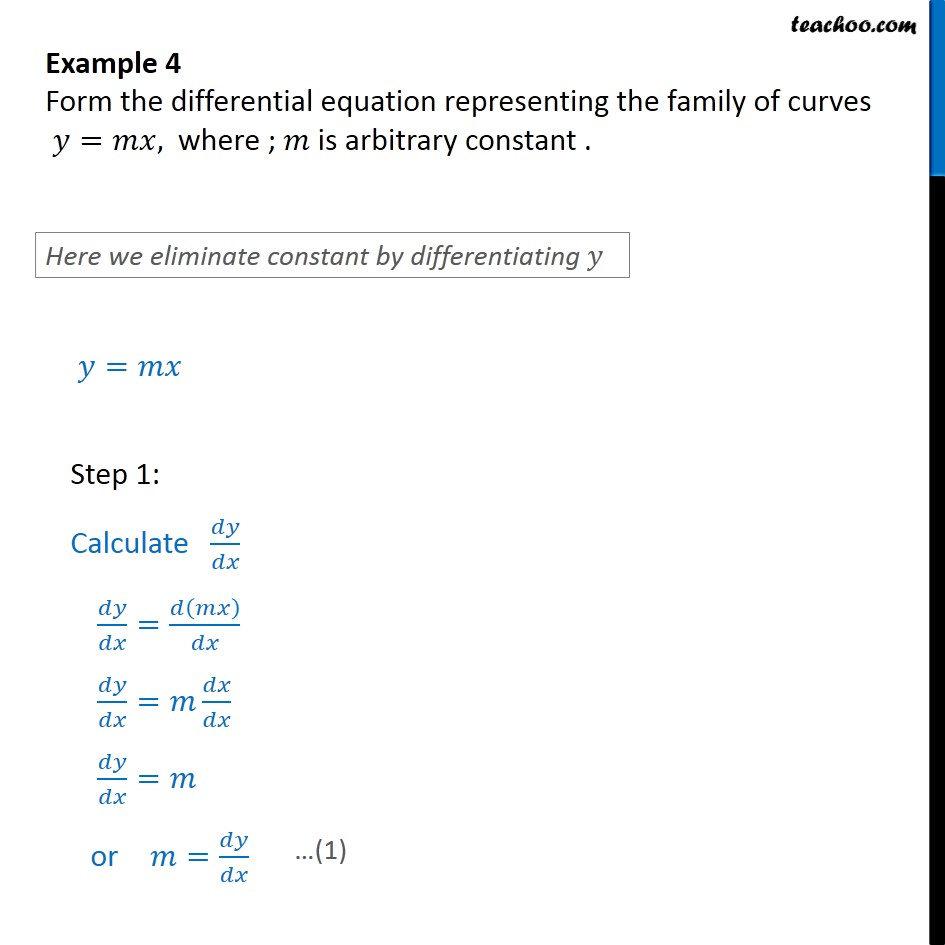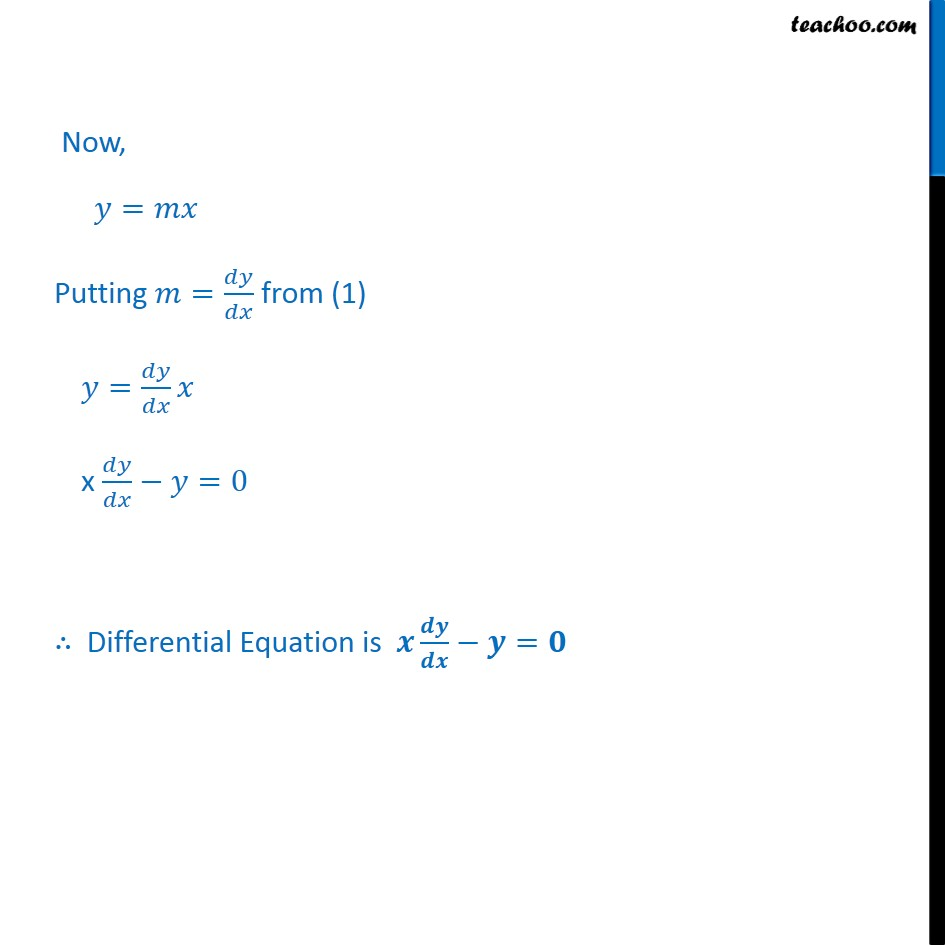Examples

Chapter 9 Class 12 Differential Equations
Serial order wiseLearn in your speed, with individual attention - Teachoo Maths 1-on-1 Class

### Transcript

Question 1 Form the differential equation representing the family of curves = , where ; is arbitrary constant . = Step 1: Calculate = = = or = Now, = Putting = from (1) = x =0 Differential Equation is =